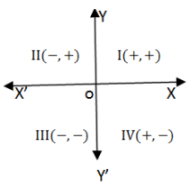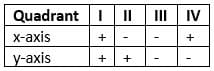Test: Coordinate Geometry- 1

# Test: Coordinate Geometry- 1

Test Description

## 10 Questions MCQ Test Quantitative Aptitude (Quant) | Test: Coordinate Geometry- 1

Test: Coordinate Geometry- 1 for Quant 2023 is part of Quantitative Aptitude (Quant) preparation. The Test: Coordinate Geometry- 1 questions and answers have been prepared according to the Quant exam syllabus.The Test: Coordinate Geometry- 1 MCQs are made for Quant 2023 Exam. Find important definitions, questions, notes, meanings, examples, exercises, MCQs and online tests for Test: Coordinate Geometry- 1 below.
Solutions of Test: Coordinate Geometry- 1 questions in English are available as part of our Quantitative Aptitude (Quant) for Quant & Test: Coordinate Geometry- 1 solutions in Hindi for Quantitative Aptitude (Quant) course. Download more important topics, notes, lectures and mock test series for Quant Exam by signing up for free. Attempt Test: Coordinate Geometry- 1 | 10 questions in 10 minutes | Mock test for Quant preparation | Free important questions MCQ to study Quantitative Aptitude (Quant) for Quant Exam | Download free PDF with solutions
 1 Crore+ students have signed up on EduRev. Have you?
Test: Coordinate Geometry- 1 - Question 1

### Q.  We divided the plane of the paper into four equal parts. by drawing two mutually perpendicular lines, X'OX and YOY'. These lines are called the axes. Here X'OX is called x-axis and YOY' is called y-axis. There axes divide the plane of the paper into four parts, called quadrants.The position of a point in a plane is denoted by an ordered pair (a,b), where a is called the x co-ordinate and y is called y co-ordinate.In which quadrant does the point(-4, -7) lie?

Detailed Solution for Test: Coordinate Geometry- 1 - Question 1

The point (-4, -7) lies in 3rd quadrant.

Test: Coordinate Geometry- 1 - Question 2

### In which quadrant does the point(1, 5) lie?

Detailed Solution for Test: Coordinate Geometry- 1 - Question 2

The point (1, 5) lies in 1st quadrant.

Test: Coordinate Geometry- 1 - Question 3

### In which quadrant does the point(9, -2) lie?

Detailed Solution for Test: Coordinate Geometry- 1 - Question 3

The point (9, -2) lies in 4th quadrant.

Test: Coordinate Geometry- 1 - Question 4

In which quadrant does the point(-7, 6) lie?

Detailed Solution for Test: Coordinate Geometry- 1 - Question 4

The point (-7, 6) lies in 2nd quadrant.

Test: Coordinate Geometry- 1 - Question 5

In which quadrant does the point(0, 9) lie?

Detailed Solution for Test: Coordinate Geometry- 1 - Question 5

The point (0, 9) lies in y-axis.

Test: Coordinate Geometry- 1 - Question 6

In which quadrant does the point(9, 0) lie?

Detailed Solution for Test: Coordinate Geometry- 1 - Question 6

The point (9, 0) lies in x-axis.

Test: Coordinate Geometry- 1 - Question 7

Find the coordinates of the point equidistant from the points A(1, 2), B(3, –4) and C(5, –6).

Detailed Solution for Test: Coordinate Geometry- 1 - Question 7

Given three points A(1,2) B(3,-4) and C(5,-6).

To find the perpendicular bisectors of AB:-

• The slope of AB:
= -4-2 / 3-1
= -3
• The slope of line perpendicular to AB:
= 1 / 3
• The mid-point of AB is:
= (1 + 3 / 2, 2 - 4 / 2)
= (2, -1)
• The equation of perpendicular line is:
y = 1 / 3(x - 2) - 1

To find the perpendicular bisectors of AC:-

• The slope of AC:
= -6 - 2 / 5 - 1
= -2
• The slope of line perpendicular to AC:
= 1 /2
• The mid-point of AC is:
= (1 + 5 / 2, 2-6 / 2)
(3, -2)
• The equation of perpendicular line is:
y = 1 / 2(x - 3) - 2

Now, solve the above two equations:-

⇒ 1 / 3(x - 2) - 1 = 1 / 2(x - 3) - 2
⇒ 2(x - 2) - 6 = 3(x - 3) - 12
⇒ x = 11
⇒ y = 1 / 2(x - 3) - 2 = 1 / 2(11 - 3) - 2 = 2

The coordinates of the points equidistant from the point A(1, 2), B(3, -4) and C(5, -6) are (11, 2)

Test: Coordinate Geometry- 1 - Question 8

Find the distance of the point A(3, -3) from the origin.

Detailed Solution for Test: Coordinate Geometry- 1 - Question 8

OA = √32+(-3)2 = √9+9 = √18 = 3√2

Test: Coordinate Geometry- 1 - Question 9

P is a point on x-axis at a distance of 4 units from y-axis to its right. The co-ordinates of P are:

Detailed Solution for Test: Coordinate Geometry- 1 - Question 9

The co-ordinates of P are A(4, 0)

Test: Coordinate Geometry- 1 - Question 10

A is a point on y-axis at a distance of 5 units from x-axis lying below x-axis. The co-ordinates of A are:

Detailed Solution for Test: Coordinate Geometry- 1 - Question 10

The co-ordinates of A are A(0, -5)

## Quantitative Aptitude (Quant)

163 videos|163 docs|131 tests
 Use Code STAYHOME200 and get INR 200 additional OFF Use Coupon Code
Information about Test: Coordinate Geometry- 1 Page
In this test you can find the Exam questions for Test: Coordinate Geometry- 1 solved & explained in the simplest way possible. Besides giving Questions and answers for Test: Coordinate Geometry- 1, EduRev gives you an ample number of Online tests for practice

## Quantitative Aptitude (Quant)

163 videos|163 docs|131 tests